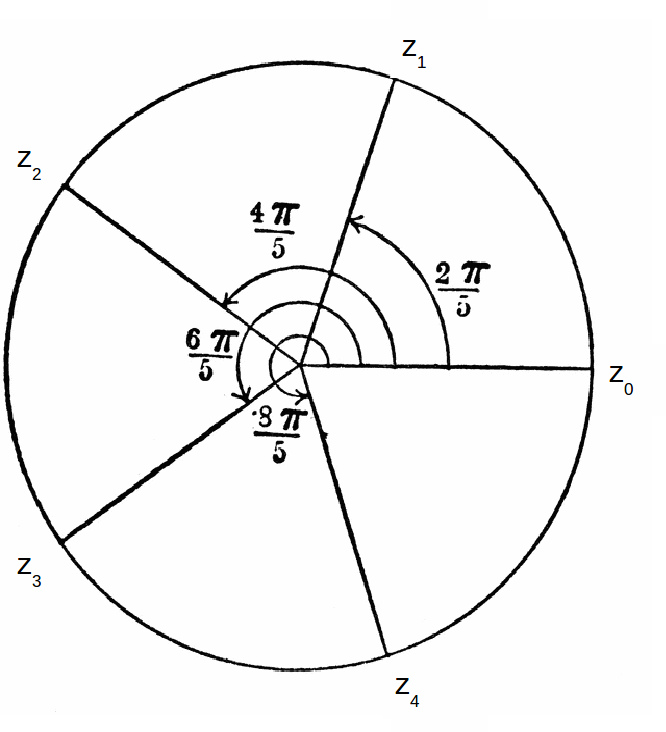## nth Roots of Unity

Finding the 'n roots of unity' means to find all solutions to the equation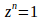(1 being unity).

We can write 1 in complex polar form as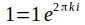where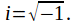Taking the nth root then gives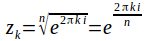For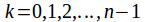the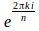are all distinct and can be plotted on an Argand diagram. The roots all lie on a circle of radius 1.

The 5 th roots of unity are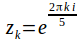for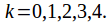These are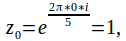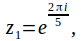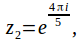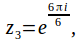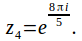These solutions are plotted on the Argand diagram below.